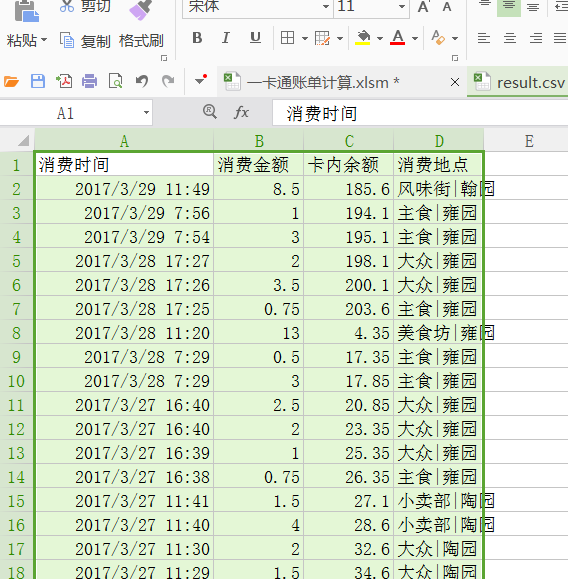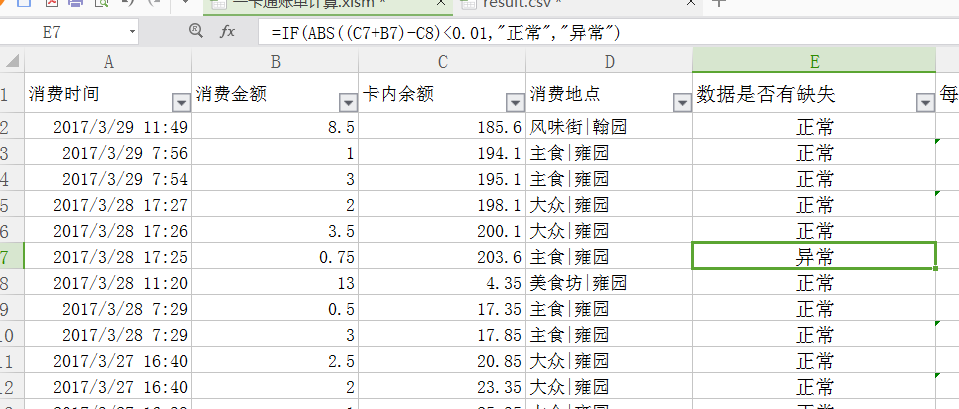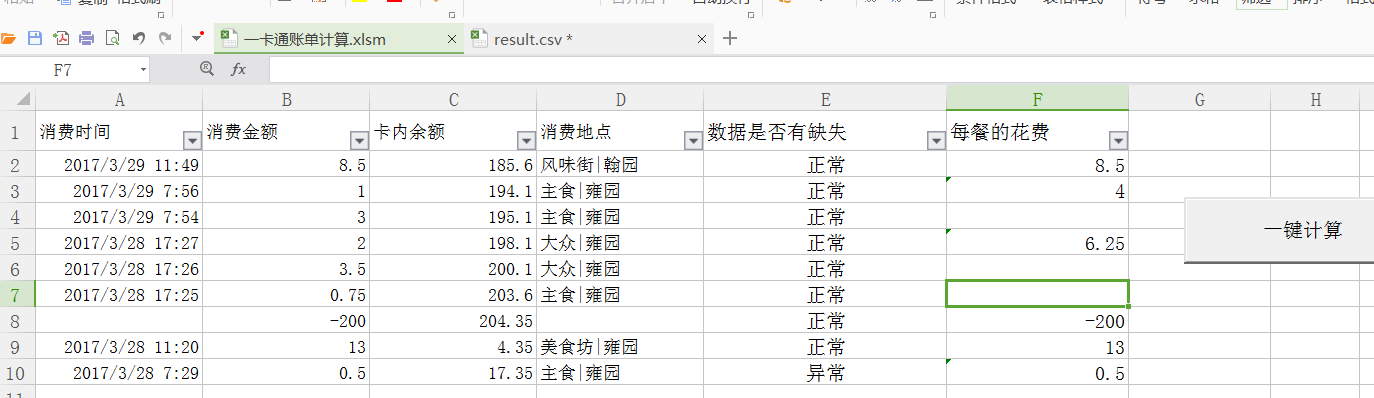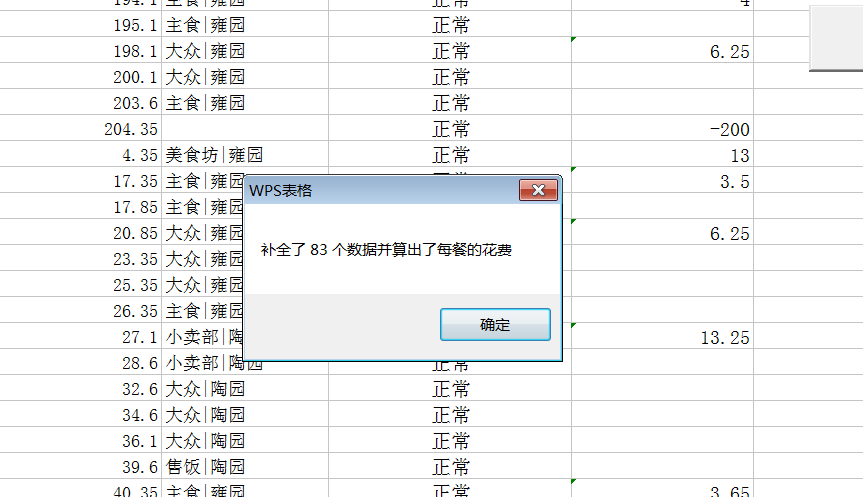1.清除文档中原有的内容2.复制result.csv的数据3.粘贴数据4.运行脚本5.计算完毕VBA代码参考

'
' 一键计算 Macro
'
' 首先删除之前的记录
Sheets("Sheet1").Range(Cells(3, 5), Cells(Sheets("Sheet1").UsedRange.Rows.Count, 5)).Clear
' 查找缺失的消费记录
Dim a, i, j, k, total
a = 0
For i = 2 To Sheets("Sheet1").UsedRange.Rows.Count
If (Abs(Sheets("Sheet1").Cells(i, 2).Value + Sheets("Sheet1").Cells(i, 3).Value - Sheets("Sheet1").Cells(i + 1, 3).Value) > 0.01) Then
'MsgBox (i)
Sheets("Sheet1").Rows(i + 1).Insert
Sheets("Sheet1").Cells(i + 1, 3).Value = Sheets("Sheet1").Cells(i, 2).Value + Sheets("Sheet1").Cells(i, 3).Value
Sheets("Sheet1").Cells(i + 1, 2).Value = (Sheets("Sheet1").Cells(i + 2, 3).Value - Sheets("Sheet1").Cells(i + 1, 3).Value)
a = a + 1
End If
Next
' 更新状态
Sheets("Sheet1").Cells(2, 5).Formula = "=IF(ABS((C2+B2)-C3)<0.01,""正常"", ""异常"")"
Sheets("Sheet1").Range("E2").Select
Selection.AutoFill Destination:=Range(Cells(2, 5), Cells(Application.WorksheetFunction.Count(Sheet1.Range("C:C")), 5)), Type:=xlFillDefault
' MsgBox ("补全了 " & a & "个数据")

'
' 计算每餐的花费 Macro
'
' 首先删除之前的记录
Sheets("Sheet1").Range(Cells(2, 6), Cells(Sheets("Sheet1").UsedRange.Rows.Count, 6)).Clear

total = Application.WorksheetFunction.Count(Sheet1.Range("C:C"))
'MsgBox (total)
For i = 2 To total
k = i
j = Cells(i, 2).Value
Do While (Abs(DateDiff("n", Cells(i, 1).Value, Cells(k + 1, 1).Value)) < 40)
k = k + 1
j = j + Cells(k, 2).Value
Loop
'MsgBox (k)
'Cells(i, 6).Value = j
Cells(i, 6).Formula = "=sum(B" & CStr(i) & ":B" & CStr(k) & ")"
i = k
Next

MsgBox ("补全了 " & a & " 个数据并算出了每餐的花费")


1.METO

啊啊啊，原来你和我就差个了中心湖的距离!

1.qing

可能是几十个中心湖的距离，我在石牌校区😂

2.iPixelOldC

其实像Python Golang这些语言都有一个很强大的excel操作库2333毕竟office的文档格式早就放出来了嘛

1.iPixelOldC

所以Ubuntu对VBA支持不好，问题应该不太大吧

2.qing

哪个顺手用哪个就好啦，VBA的话主要是要Excel有微软的引擎才能解析

3.Kay

消费不到1元的你到底消费的是啥

1.qing

先在售饭窗口点饭（这里打一次卡），然后再到别的窗口点菜（再打若干次卡），所以就这样子啦